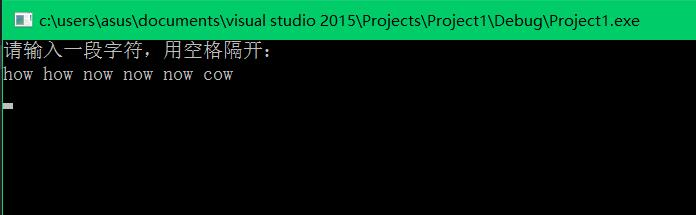#include
#include
#include

using namespace std;

int main()
{
string currString, preString = "", maxString;
int currcnt = 1, maxcnt = 0;
cout << "请输入一段字符，用空格隔开：" << endl;
while (cin >> currString)
{
if (currString == preString)
{
++currcnt;
if (currcnt > maxcnt)
{
maxcnt = currcnt;
maxString = currString;
}
}
else
{
currcnt = 1;
}
preString = currString;
}
if (maxcnt > 1)
cout << "出现最多的字符串是：" << maxString << ",次数是" << maxcnt << endl;
else
cout << "每个字符串都出现了一次" << endl;
system("pause");
return 0;
}

2个回答

`````` while (cin >> currString)

http://blog.csdn.net/zhoujunbuaa/article/details/5975237
``````3 年多之前 回复3 年多之前 回复